Topics: AI | Data Science | Data Visualization | Deep Learning | Machine Learning | NLP | Python | R | Statistics

KDnuggets Home » News » 2020 » May » Tutorials, Overviews » An easy guide to choose the right Machine Learning algorithm ( 20:n21 )

#An easy guide to choose the right Machine Learning algorithm

There's no free lunch in machine learning. So, determining which algorithm to use depends on many factors from the type of problem at hand to the type of output you are looking for. This guide offers several considerations to review when exploring the right ML approach for your dataset.

By Yogita Kinha, Consultant and Blogger.Well, there is no straightforward and sure-shot answer to this question. The answer depends on many factors like the problem statement and the kind of output you want, type and size of the data, the available computational time, number of features, and observations in the data, to name a few.

Here are some important considerations while choosing an algorithm.

### 1. Size of the training data

It is usually recommended to gather a good amount of data to get reliable predictions. However, many a time, the availability of data is a constraint. So, if the training data is smaller or if the dataset has a fewer number of observations and a higher number of features like genetics or textual data, choose algorithms with high bias/low variance like Linear regression, Naïve Bayes, or Linear SVM.

If the training data is sufficiently large and the number of observations is higher as compared to the number of features, one can go for low bias/high variance algorithms like KNN, Decision trees, or kernel SVM.

### 2. Accuracy and/or Interpretability of the output

Accuracy of a model means that the function predicts a response value for a given observation, which is close to the true response value for that observation. A highly interpretable algorithm (restrictive models like Linear Regression) means that one can easily understand how any individual predictor is associated with the response while the flexible models give higher accuracy at the cost of low interpretability.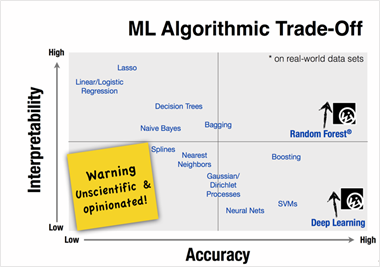A representation of trade-off between Accuracy and Interpretability, using different statistical learning methods. (source)

Some algorithms are called Restrictive because they produce a small range of shapes of the mapping function. For example, linear regression is a restrictive approach because it can only generate linear functions such as the lines.

Some algorithms are called flexible because they can generate a wider range of possible shapes of the mapping function. For example, KNN with k=1 is highly flexible as it will consider every input data point to generate the mapping output function. The below picture displays the trade-off between flexible and restrictive algorithms.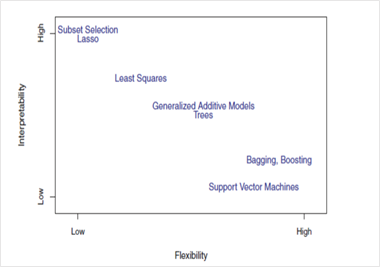A representation of trade-off between Flexibility and Interpretability, using different statistical learning methods. (source)

Now, to use which algorithm depends on the objective of the business problem. If inference is the goal, then restrictive models are better as they are much more interpretable. Flexible models are better if higher accuracy is the goal. In general, as the flexibility of a method increases, its interpretability decreases.

### 3. Speed or Training time

Higher accuracy typically means higher training time. Also, algorithms require more time to train on large training data. In real-world applications, the choice of algorithm is driven by these two factors predominantly.

Algorithms like Naïve Bayes and Linear and Logistic regression are easy to implement and quick to run. Algorithms like SVM, which involve tuning of parameters, Neural networks with high convergence time, and random forests, need a lot of time to train the data.

### 4. Linearity

Many algorithms work on the assumption that classes can be separated by a straight line (or its higher-dimensional analog). Examples include logistic regression and support vector machines. Linear regression algorithms assume that data trends follow a straight line. If the data is linear, then these algorithms perform quite good.

However, not always is the data is linear, so we require other algorithms which can handle high dimensional and complex data structures. Examples include kernel SVM, random forest, neural nets.

The best way to find out the linearity is to either fit a linear line or run a logistic regression or SVM and check for residual errors. A higher error means the data is not linear and would need complex algorithms to fit.

### 5. Number of features

The dataset may have a large number of features that may not all be relevant and significant. For a certain type of data, such as genetics or textual, the number of features can be very large compared to the number of data points.

A large number of features can bog down some learning algorithms, making training time unfeasibly long. SVM is better suited in case of data with large feature space and lesser observations. PCA and feature selection techniques should be used to reduce dimensionality and select important features.

Here is a handy cheat sheet that details the algorithms you can use for different types of machine learning problems.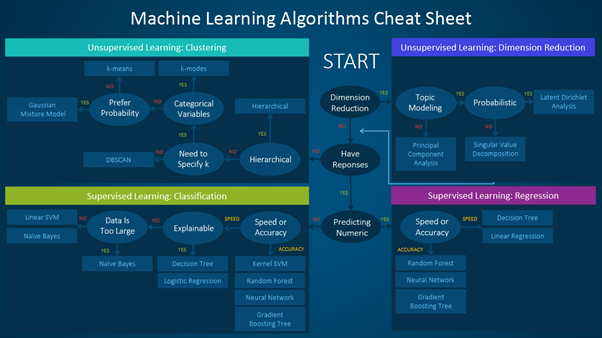source

Machine learning algorithms can be divided into supervised, unsupervised, and reinforcement learning, as discussed in my previous blog. This article walks you through the process of how to use the sheet.

The cheat sheet is majorly divided into two types of learning:

Supervised learning algorithms are employed where the training data has output variables corresponding to the input variables. The algorithm analyses the input data and learns a function to map the relationship between the input and output variables.

Supervised learning can further be classified into Regression, Classification, Forecasting, and Anomaly Detection.

Unsupervised Learning algorithms are used when the training data does not have a response variable. Such algorithms try to find the intrinsic pattern and hidden structures in the data. Clustering and Dimension Reduction algorithms are types of unsupervised learning algorithms.

The below infographic simply explains Regression, classification, anomaly detection, and clustering along with examples where each of these could be applied.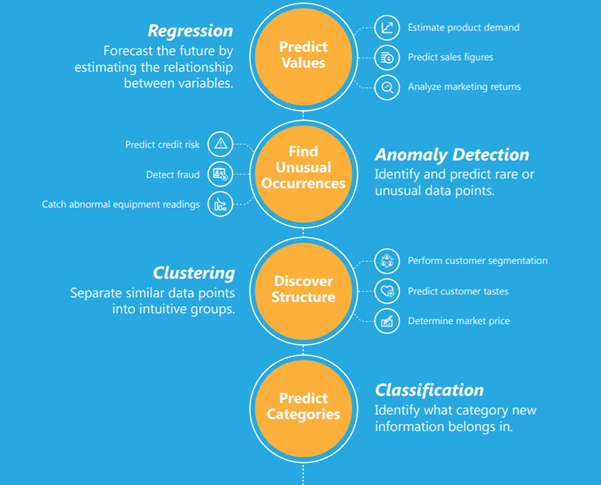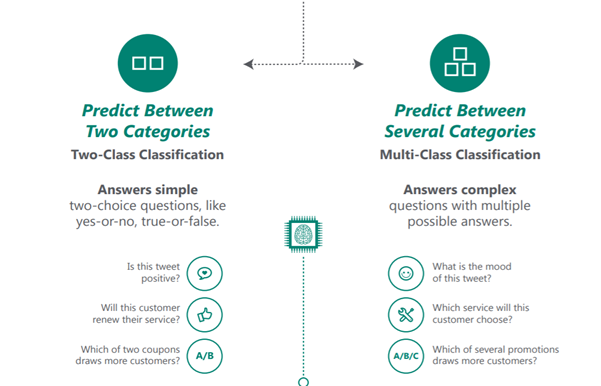source

The main points to consider when trying to solve a new problem are:

• Define the problem. What is the objective of the problem?
• Explore the data and familiarise yourself with the data.
• Start with basic models to build a baseline model and then try more complicated methods.

Having said that, always remember that “better data often beats better algorithms,” as discussed in my previous blog. Equally important is designing good features. Try a bunch of algorithms and compare their performances to choose the best one for your specific task. Also, try ensemble methods as they generally provide much better accuracy.

Original. Reposted with permission.

Related:

Top Stories Past 30 Days
Most Popular
Most SharedGet KDnuggets, a leading newsletter on AI, Data Science, and Machine Learning